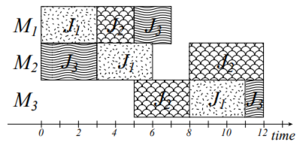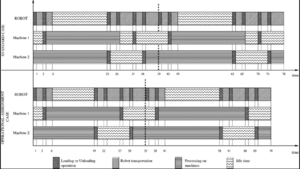# Difference between revisions of "Job shop scheduling"

Authors: Carly Cozzolino, Brianna Christiansen, Elaine Vallejos, Michael Sorce, Jeff Visk (SYSEN 5800 Fall 2021)

## Introduction

The Job-Shop Scheduling Problem (JSSP) is a combinatorial optimization problem, which mainly concerns the operations industry. The aim of the problem is to optimize the schedule for allocation of shared resources over time to competing activities.

Within a JSSP you will have n number of jobs (J1, J2, ..., Jn ), that will need to be completed using a m number shared resources, most commonly denoted as machines (M1, M2, ..., Mm ). Each job will have operations (O) that will need to be completed in a specific order in order for the job to be completed. Operations must be completed at specific machines and require a specific amount of processing time (p) on that machine.Machines are assumed to only be able to process one operation at a time, driving the need to optimize the order in which these operations are completed. The goal of the JSSP is to minimize the overall time it takes to complete all n jobs within the problem.

## Theory/Methodology/AlgorithmsFigure 1: A Gantt-Chart representation of the job shop scheduling problem

The job shop scheduling problem is NP-hard meaning it’s complexity class for non-deterministic polynomial-time is least as hard as the hardest of problems in NP. As an input, there is a finite set J of jobs and a finite set of M of machines. Some jobs may need to be processed through multiple machines giving us an optimal potential processing order for each job. The processing time of the job j on machine m can be defined as the nonnegative integer . In order to minimize total completion time, the job shop can find the optimal schedule of J on M.

The assumptions made for solving the problem are as follows. The number of operations for each job is finite. The processing time for each operation in a particular machine is defined. There is a pre-defined sequence of operations that has to be maintained to complete each job. Delivery times of the products are undefined. No setup cost and tardiness cost. A machine can process only one job at a time. Each job visits each machine only once. No machine can deal with more than one type of task. The system cannot be interrupted until each operation of each job is finished. No machine can halt a job and start another job, before finishing the previous one. Given these assumptions, the problem can be solved at complexity class NP-hard.

One way of visualizing the job shop scheduling problem is using a Gantt-Chart. Given a problem of size 3 x 3 (J x M), a solution can be represented as shown in Figure 1. Depending on the job, the operating time on each machine could vary.

### Methods: Branch and Bound

One of the most common problem solving methods for the Job Shop Scheduling Problem is the Branch and Bound method.  The first step is to define the decision variables, specifying that Xij is equal to 1 given that job j is placed in the ith position. Outside of that stipulation, Xij is equal to 0. The jobs are referred to as A, B, and C. The total delay, T, is equal to delay 1 plus delay 2 plus delay 3.The durations and due dates must be specified to produce a solvable problem. Each branch or option will be explored to find the most minimized delay time and thus the most optimized job sequence. Thus we have:

Xij = 1

Xij = 0

i = 1, 2, 3

J = A, B, C

T = d1 + d2 + d3

Initially, the delay times, the total delay, or the position of any of the jobs are all unknown. The Branch and Bound method sets out to solve for each of those to arrive at the optimal sequence for which the jobs need to be performed to minimize the total delay time assuming the completion of all the jobs.

Starting with the last position, where i = 3, suppose X3a = 1 by placing A in the third position, then suppose X3b = 1 by placing B in the third position, and lastly suppose X3c = 1 by placing C in the third position.

Starting with X3a = 1, no matter what the first 2 positions sum to, it is known that the third position will always be completed on the final day, which is computed by adding all the durations together. Next the delay is calculated by subtracting the due date of job A from the total duration; this is delay 3. This states then that the total delay time is less than or equal to d3. This same process can be used to calculate d3 for both of the other branch (X3b = 1 and X3c = 1).

Next, take the case which provided the smallest total delay value since this is the most likely to provide the shortest delay time. Assuming this case, the third position is decided. This does not guarantee the shortest position, however, so the other methods may need to be calculated as well. This branch is extended by checking the second position. Position 2 could now be one of the remaining two positions, let's assume option A and B remain. Set X2a = 1 and X2b =1 respectively for each branch. For X2a = 1, take the total duration and subtract the duration of the selected job for I = 3, (C in this case) from the total. Subtract the due date of job A from that calculated value to get d2. The total delay must then be greater than or equal to d2 plus d3. Repeat this process for X2b = 1.

The branch with the smaller total delay time is likely the minimized solution, but this option needs to be checked by placing the remaining job in the first position. To do this, calculate the completion time of the job when i = 1 by taking the total duration of the jobs summed and subtracting the duration of the job when i = 3 and when i = 2. To calculate d1, take the due date of the job when i = 1 and subtract the completion time of the that job. Then sum d1, d2, and d3 to calculate the total delay, T.

Lastly, ensure each total delay time is not less than previously found. If one of the other branches has a value that is less than or equal to the calculated T, follow this same process to check the other 2 initial branches. The smallest T shows the optimal solution and the sequence utilized to provide the minimized delay time.

## Job-Shop Scheduling - CNC Machine Numerical Example

This example demonstrates how a Job-Shop Scheduling problem can be solved using the Branch and Bound method explained above.

There are three parts that need to be processed (jobs) on a single available CNC machine. Each job has a finite duration to complete and a due date in the overall project schedule. This information is shown in the following table:

CNC Part Processing Information
Job Duration (days) Due Date
A 6 Day 8
B 4 Day 4
C 5 Day 12

The goal of this problem is to determine which sequence the jobs should be completed in to minimize total accrued program delay. The following table shows an example to calculate delay for one particular sequence.

Delay for Sequence (A, B, C)
Job Completion Day Delay
A 6 d1 = 6-8 = -2 -> 0
A, B 6+4 = 10 d2 = 10- 4 = 6
A, B, C 6+4+5 d3 = 15 - 12 = 3

As you can see by beginning with task A we will have that completed by day 6. This is 2 days before the day 8 deadline and adds no delay to our overall schedule. At completion of task B we add the time it takes for A and B to complete to get the

## Applications

There are several ways in which the job-shop scheduling problem can be modified, often to simplify the problem at hand, for a variety of applications.

The most obvious example can be found in the manufacturing industry, as the name suggests. It is common for some production jobs to require certain machines to perform tasks, due to the proper capabilities or equipment of a given machine. This adds an additional layer of complexity to the problem, because not any job can be processed on any machine. This is known as flexible manufacturing. Furthermore, there are many uncertain factors that are not accounted for in the basic understanding of the problem, such as delays in delivery of necessary supplies, significant absence of workers, equipment malfunction, etc. 

This problem can also be applied to many projects in the technology industry. In computer programming, it is typical that computer instructions can only be executed one at a time on a single processor, sequentially. In this example of multiprocessor task scheduling, the instructions can be thought of as the "jobs" to be performed and the processors required for each task can be compared to the "machines". Here we would want to schedule the order of instructions such that the number of operations performed is maximized to make the computer as efficient as possible. Within this application, there are a further variety of heuristic methods considered as to how to execute tasks to maximize efficiency and minimize costs, including minimum completion time, duplex, max-min, min-min, tabu search, and genetic algorithm. Each of these methods have their advantages and disadvantages, weighing the importance of minimizing execution time versus finding the closest to optimal solution.Fig 2. Comparison of Gantt charts for the standard and operational assignment cases, two common methods of robot move cycles.

With the progression of automation in recent years, robotic tasks such as moving objects from one location to another are similarly optimized. In this application, the extent of movement of the robot is minimized while conducting the most amount of transport jobs to support the most effective productivity of the robot. There are additional nuances within this problem as well, such as the rotational movement capabilities of the robot, the layout of the robots within the system, and the number of parts or units the robot is able to produce. While each of these factors contribute to the complexity of the job shop scheduling problem, they are all worth considering as more and more companies are proceeding in this direction to reduce labor costs and increase flexibility and safety of the enterprise.

## Conclusions

Job-Shop Scheduling Problems are NP-hard combinatorial optimization problems, predominately used for operations applications. JSSPs minimize the overall time a set of jobs takes to complete, by optimizing the schedule in which shared resources are set to be allocated.

JSSPs can be solved using a variety of methods and algorithms.

There are multiple applications for JSSPs, the predominate application is within the manufacturing industry as the name implies. Other applications for this problem could also be the optimization of a computer's processing power as it executes multiple programs, optimization of automated equipment or robots, as well as a number of other applications.